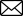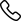# How to Calculate Total Interest Paid on a Car Loan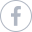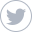Find out if you’re getting ripped off on your car insurance in less than two minutes.
No long forms · No spam · No fees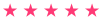3.5k Ratings
When applying for a car loan, you first need to determine if the monthly payment fits into your budget. A big part of financing the purchase of a new car includes the interest you pay the lender for letting you borrow the money in the first place. You can easily calculate the total interest you need to pay by using a simple formula.
Luckily, car insurance and broker app Jerry has you covered on all the basics to figure out exactly how you can finance your car.

## How to determine the principal, loan term, and the interest rate on your car loan

### Step 1: Determine the principal

The principal owed represents the amount of money you want to finance. The principal includes the total amount you have to pay for the car minus any rebates, the value of any vehicles you trade in, and the amount of the down payment. In addition, you need to add any dealer fees, sales tax, and other fees associated with buying a car.

### Step 2: Determine the loan term

The term of the loan can range from 24 months to 84 months. The longer the loan term, the more interest you pay. Also, paying off a car loan early usually results in penalties from the lender, so check before doing so.

### Step 3: Determine the interest rate

To find the interest rate on the car loan you plan on applying for, check out the loan agreement.
The interest rate applies to how much you actually borrow plus any taxes. You can find the Annual Percentage Rate (APR) on the disclosure form section of the loan agreement you have to sign when applying for a car loan.

## How to calculate the total interest paid on a car loan

### Step 1: The amortization loan formula

Once you know how much you want to borrow and the interest rate that you need to apply, you can use a simple formula to calculate how much interest you can expect to pay over the life of the loan. To determine how much in interest you can expect to pay, use the following amortization calculation formula:
A = P x (r(1+r)n)/((1+r)n - 1)
In this formula the following signifies certain factors:
• A = the monthly payment
• P = the principal balance
• r = the interest rate per month
• n = the lease term length in months

### Step 2: Determine the monthly interest rate

Start by dividing the yearly APR by 12. This gives you the amount of interest charged on a monthly basis.

### Step 3: Determine the first part of the formula

Next, for the first part of the equation, or (r(1+r)n), add one to the interest rate per month.
Then enter this number and press the exponent button on the calculator, denoted as x^y. After pressing the exponent button, enter the number corresponding to the number of months of the loan.
Finally, multiply the exponent by the amount of the monthly APR, which gives you a decimal sum.

### Step 4: Determine the second part of the formula

For the next part of the formula, or ((1+r)n - 1), add 1 to the APR per month and then get the exponent by pressing the exponent button, or x^y, and the number of months of the loan term. Subtract 1 from this total to get a digital sum.

### Step 5: Determine total amount due

Divide the first sum by the second sum.
Multiply the amount gained by the total amount of the principal, giving you the payment per month.
Multiply the monthly payment amount by the number of months of the loan to get the total amount you have to pay back over the loan term, including interest.

### Step 6: Determine total interest paid on your car

Finally, subtract the principal from the total amount you have to pay back to get the total interest amount.
The ability to figure out how much you need to pay in interest over the course of a car loan term allows you to budget appropriately. It also allows you to compare different loan amount and choose the car that best fits your needs and falls within your budget.

## FAQs

### Why do I need to calculate total interest on loan?

It’s always important to keep track of your finances when buying a car. Vehicles are expensive investments, so you need to know what you’re getting yourself into. It’s also possible that there could be hidden fees or terms in your loan agreement, so running the numbers yourself is always preferable.

### Where can I find cheap car insurance?

If you’re trying to cut costs on your car loan, you might want to do so elsewhere, too. And saving a lot on car insurance has never been easier. To get the best rate for you, try Jerry. Jerry is the easiest and most effective way to find a car insurance policy that is customized for you.
After providing you with a comprehensive cross-analysis of the best policies across providers, Jerry will handle the phone calls, paperwork, and renewals for your top pick so that you don’t have to.

# Easiest way to compare and buy car insurance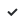No long formsNo spam or unwanted phone callsQuotes from top insurance companies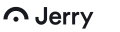JERRY INSURANCE AGENCY is a licensed insurance agency in 50 states.